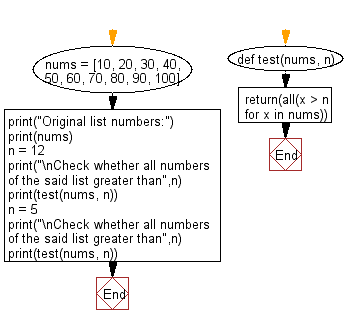﻿ Python: Test whether all numbers of a list is greater than a certain number - w3resource# Python: Test whether all numbers of a list is greater than a certain number

## Python Basic: Exercise-83 with Solution

Write a Python program to test whether all numbers of a list is greater than a certain number.

Pictorial Presentation:Sample Solution-1:

Python Code:

``````num = [2, 3, 4, 5]
print()
print(all(x > 1 for x in num))
print(all(x > 4 for x in num))
print()
```
```

Sample Output:

```True
False
```

## Visualize Python code execution:

The following tool visualize what the computer is doing step-by-step as it executes the said program:

Sample Solution-2:

Python Code:

``````def test(nums, n):
return(all(x > n for x in nums))
nums = [10, 20, 30, 40, 50, 60, 70, 80, 90, 100]
print("Original list numbers:")
print(nums)
n = 12
print("\nCheck whether all numbers of the said list greater than",n)
print(test(nums, n))
n = 5
print("\nCheck whether all numbers of the said list greater than",n)
print(test(nums, n))
```
```

Sample Output:

```Original list numbers:
[10, 20, 30, 40, 50, 60, 70, 80, 90, 100]

Check whether all numbers of the said list greater than 12
False

Check whether all numbers of the said list greater than 5
True
```

Flowchart:## Visualize Python code execution:

The following tool visualize what the computer is doing step-by-step as it executes the said program:

Python Code Editor:

Have another way to solve this solution? Contribute your code (and comments) through Disqus.

What is the difficulty level of this exercise?

Test your Python skills with w3resource's quiz

﻿

## Python: Tips of the Day

Try-catch-else construct:

```try:
foo()
except Exception:
print("Exception occured")
else:
print("Exception didnt occur")
finally:
print("Always gets here")
```